## LetsPlayMaths.Com

WELCOME TO THE WORLD OF MATHEMATICS

# Class 3 Division

Class II Recap

Division by a One digit Number Without Remainder

Division by a One digit Number With Remainder

Division by 10 and 100

Division by Two digit Number

Division by Three digit Number

Story Time Division

Division Test

Division Worksheet

## Class II Recap

In class II, we have learnt that division is nothing but repeated subtraction. In other word, we can say division is a process by which equal amount of distribution can be done among a group.
Few examples are given below.

Example 1. 48 ÷ 6 = __________

Solution.We must recall the 6 tables and find out where 48 comes. That is 6 X 8 = 48.
So, the answer will be 8.

Example 2. 36 ÷ 18 = _________

Solution. We must recall 18 tables and find out where 36 comes. That is 18 X 2 = 36.
So, the answer will be 2.

Some of the basic Division properties are given below.

1. When a number is divided by itself then the quotient will be 1. That means, 7 ÷ 7 = 1

2. When a number is divided by 1 then the quotient will be the number itself.That means, 9 ÷ 1 = 9

3. When 0 is divided by any number then the quotient will be 0 itself. That means, 0 ÷ 5 = 0

## Division by a One digit Number Without Remainder

We have learnt division of 3 digit numbers by 1 digit number. We will use same method for division of 4 or 5 digit numbers by 1 digit numbers without remainder. Few examples are given below.

Example 1. Divide 4242 by 2.

Solution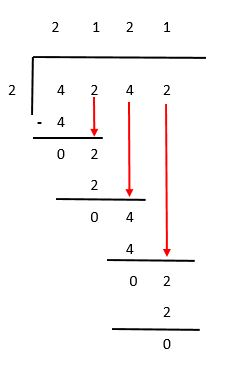First divide thousands place.

4 thousands ÷ 2 = 2 thousands

We write 2 in thousands place in the quotient.

Next divide hundreds place

2 hundreds ÷ 2 = 1 hundreds

We write 1 in hundreds place in the quotient.

Next divide tens place

4 tens ÷ 2 = 2 tens

We write 2 in tens place in the quotient.

Next divide ones place

2 ones ÷ 1 = 1 ones

We write 1 in once place in the quotient.

So, 4242 ÷ 2 = 2121.

Example 2. Divide 36252 by 3

Solution.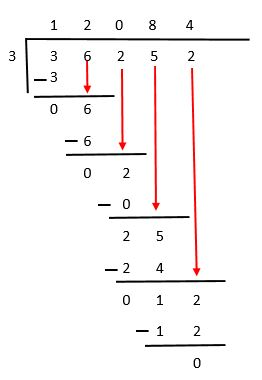First divide the ten-thousands place

3 ten-thousands ÷ 3 = 1 ten-thousands

So, write 1 in the ten-thousands place in the quotient.

Next divide thousands place

6 thousands ÷ 3 = 2 thousands

So, write 2 in the thousands place in the quotient.

Next divide hundreds place

2 hundreds ÷ 3 = 0 hundreds

So, write 0 in the hundreds place in the quotient. Subtract zero hundred from 2 hundred,

remainder is 2 hundreds

Next divide 25 tens by 3

25 tens ÷ 3 = 8 tens

So, write 8 in the tens place in the quotient. Subtract 24 tens from 25 tens and get 1 tens as remainder.

Now, 1 tens and 2 ones makes 12 ones

Next divide 12 ones by 3

12 ones ÷ 3 = 4 ones

So, write 4 in the ones place of quotient and subtract 3 X 4 = 12 ones from 12 ones. Remainder in 0.

Thus 36252 ÷ 3 = 12084

Let’s take some example where first digit on the left most of the dividend is not divisible by the divisor.

Example 3. Divide 1575 by 5

Solution.Left most digit of the dividend is 1 and it is lesser than divisor that is 5. Therefore,

we must consider hundreds place. Now 1 thousand and 5 hundreds is equal to 15 hundreds.

15 hundreds ÷ 5 = 3 hundreds

So, write 3 in the hundreds place of the quotient and subtract 15 from 15 leaving 0.

Now, bring down 7 tens from the dividend as shown in the above figure.

7 tens ÷ 5 = 1 tens 2 remainder

So, write 1 in the tens place of quotient and subtract 5 from 7 leaving 2.

Now bring 5 ones from the dividend.

25 ones ÷ 5 = 5 Ones

So, write 5 in the ones place of quotient and subtract 25 from 25 leaving 0 as remainder.

Example 4. Divide 52402 ÷ 7

Solution.Left most digit of dividend is 5 and it is lesser than divisor that is 7. Therefore,

we must consider thousands place. Now 5 ten-thousands and 2 thousands is equal to 52 thousands.

52 Thousands ÷ 7 = 7 Thousands as quotient and 3 as remainder. So, write 7 in the thousands place of quotient.

Now, bring down 4 hundreds from the dividend to the right of remainder 3 as shown in the above

figure. We have 34 hundreds with us now.

34 hundreds ÷ 7 = 4 hundreds as quotient and 6 hundred as remainder.

Now, bring down 0 tens from the dividend to the right of remainder 6 as shown above.

60 tens ÷ 7 = 8 tens as quotient and 4 tens as remainder.

Now, bring down 2 ones from the dividend to the right of remainder 4 as shown above.

42 ones ÷ 7 = 6 Ones as quotient and 0 remainder.

Thus, 52402 ÷ 7 = 7486

## Division by a One digit Number With Remainder

Let’s assume we have 6 chocolates and we must divide it among 5 children equally. If we give one chocolate to each child, then 5 chocolates will be over, and 1 chocolate will remain with us. Let’s go through some examples given below.

Example 1. Divide 4527 by 5

Solution. Use the division rules explained earlier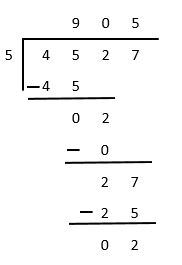So, 4527 ÷ 5 = 905 is Quotient and 2 is Remainder

Example 2. Divide 27315 by 7

Solution. Arrange the dividend and divisor in a tabular format as given in below figureSo, 27315 ÷ 7 = 3902 is Quotient and 1 is remainder

Rules to verify the division
1. Division without remainder

Dividend = Quotient X Divisor

2. Division with remainder

Dividend = (Quotient X Divisor) + Remainder

## Division by 10 and 100

When we divide a number by 10, the digit at the ones place of the dividend is the remainder. The number formed by the digits after removing the ones place digit of the dividend is the quotient.

Let’s see some examples.

Example 1. Divide 8500 by 10

Solution.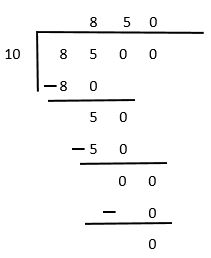Quotient = 850

Remainder = 0

Example 2. Divide 96758 by 10

Solution.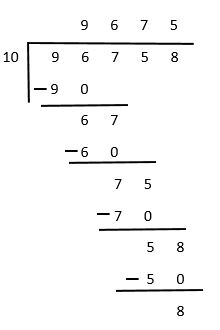Quotient = 9675

Remainder = 8

When we divide a number by 100, the number formed by tens and ones place of the dividend is the reminder. The number formed by remaining digits of dividend is quotient.

Let’s go through some of the examples.

Example 1. Divide 3057 by 100.

Solution.Quotient = 30

Remainder = 57

Example 2. Divide 45705 by 100

Solution.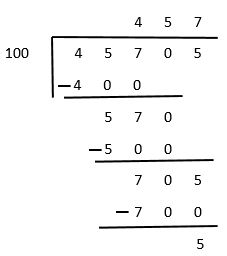Quotient = 457

Remainder = 5

## Division by Two digit Number

All the rules that we have learnt earlier will be used in this kind of division. Here, we will have 2 digits divisor. Let’s see some examples.

Example 1. Divide 1740 by 15

Solution.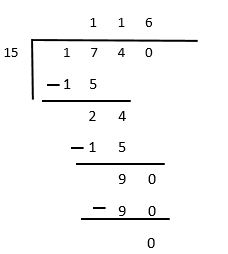15 is the divisor here. The two digits on the extreme left of the dividend is 17.

17 is greater than 15.

17 ÷ 15 = 1 Quotient and 2 is Remainder.

1 goes to the hundreds place of quotient as shown in the figure. 4 came down and sat on

the right side of the remainder 2. Now new dividend is 24.

24 ÷ 15 = 1 Quotient and 9 is Remainder.

1 goes to the tens place of quotient. 0 came down and sat on the right side of the remainder 9.

Now the new dividend is 90.

90 ÷ 15 = 6 Quotient and 0 is Remainder.

6 goes to the ones place of quotient.

Thus, 1740 ÷ 15 = 116

Example 2. Divide 16985 by 20

Solution.Here the divisor is 20. The two digits number on the extreme left of the dividend is 16

and it is less than 20. So, we consider 3 digits number from the dividend that is 169.

169 ÷ 20 = 8 Quotient and 9 Remainder

Write 8 in the hundreds place of quotient. Bring down 8 from the dividend and place it on the

right side of reminder 9. Now, the new dividend is 98.

98 ÷ 20 = 4 Quotient and 18 remainder

Write 4 in the tens place of quotient. Bring down 5 from the dividend and place it on the right

side of remainder 18. Now, the new dividend is 185.

185 ÷ 20 = 9 Quotient and 5 remainder

Write 9 in the ones place of quotient and 5 in the remainder

Thus, 16985 ÷ 20 = 849 Quotient and 5 Remainder

## Division by Three digit Number

All the rules that we have learnt earlier will be used in this kind of division. Here, we will have 3 digits divisor. Let’s see some examples.

Example 1. Divide 6708 by 120

Solution.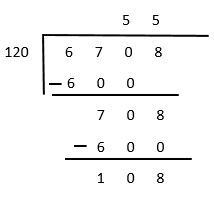Here the divisor has 3 digits. The three digits number on the extreme left of the dividend is 670.

So, the dividend is 169 and divisor is 120.

670 ÷ 120 = 5 Quotient and 70 Remainder

Write 5 in the tens place of quotient. Bring down 8 from the dividend and place it on the right

side of reminder 70. Now, the new dividend is 708.

708 ÷ 120 = 5 Quotient and 108 remainder

Write 5 in the ones place of quotient.

Thus, 6708 ÷ 120 = 55 Quotient and 108 remainder

Example 2. Divide 12359 by 185

Solution.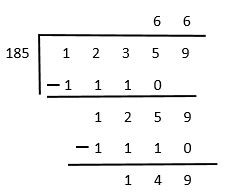Here the divisor has 3 digits. The three digits number on the extreme left of the dividend is 123.

But, 123 is less than 185. So, we have to consider one more digit from right. Now the new dividend is 1235.

1235 ÷ 185 = 6 quotient and 125 remainder

Write 6 in the tens place of quotient. Bring down 9 from the dividend and place it on the right side of

reminder 125. Now, the new dividend is 1259.

1259 ÷ 185 = 6 Quotient and 149 remainder

Write 6 in the ones place of quotient.

Thus, 12359 ÷ 185 = 66 Quotient and 149 remainder

## Story Time Division

In our day to day life we use concept of multiplication in many ways, few examples are shown below.

Example 1. There are 9 students in a classroom, and the class teacher has 2457 chocolates with him. How many chocolates each student will get?

Solution.In order to find the number of chocolates each student will get, we have to divide 2457 by 9.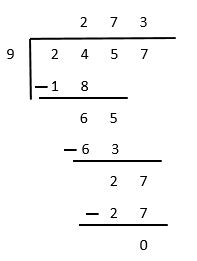Thus, each student will get 273 chocolates.

Example 2. 8 houses cost 98496 dollars, and all the houses are of same cost. what is the cost of each house?

Solution.To get the price of each house, we must divide 98496 by 8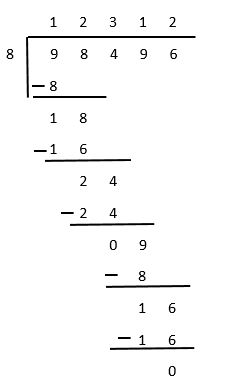Thus, cost of each house is 12312 dollars

Example 3. A shopkeeper bought some dresses each costing 12 dollars. He gave 54820 dollars to the manufacturer. How many dresses did he buy and what balance did he get back?

Solution.Shopkeeper bought 4568 dresses and he has 4 dollars balance with him.

## Division Test

Division Test - 1

Division Test - 2

## Division Worksheet

Division Worksheet - 1

Division Worksheet - 2

Division Worksheet - 3